# Performing Calculations Using Functions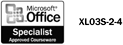Functions are predesigned formulas that save you the time and trouble of creating commonly used or complex equations. Excel includes hundreds of functions that you can use alone or in combination with other formulas or functions. Functions perform a variety of calculations, from adding, averaging, and counting to more complicated tasks , such as calculating the monthly payment amount of a loan. You can enter a function manually if you know its name and all the required arguments, or you can easily insert a function using the Paste Function feature.

#### Enter a Function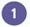Click the cell where you want to enter the function.Type = (an equal sign), type the name of the function, and then type ( (an opening parenthesis). For example, to insert the AVERAGE function, type =AVERAGE( .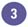Type the argument or select the cell or range you want to insert in the function.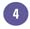Click the Enter button on the formula bar, or press Enter.

Excel will automatically add the closing parenthesis to complete the function.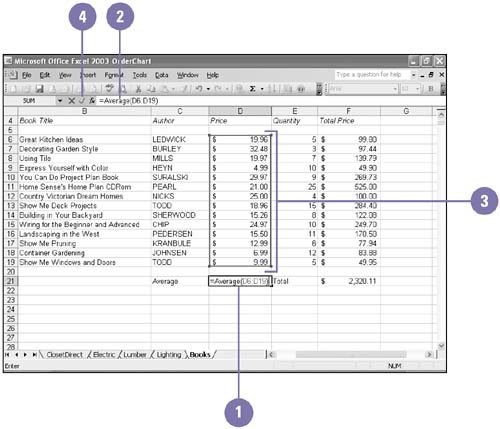See " Displaying Calculations with AutoCalculate " on page 65 for information on getting the results of a simple calculation quickly.

## Commonly Used Excel Functions

Function

Description

Sample

SUM

Displays the sum of the argument

=SUM(argument)

AVERAGE

Displays the average value in the argument

=AVERAGE(argument)

COUNT

Calculates the number of values in the argument

=COUNT(argument)

PMT

Determines the monthly payment of a loan

=PMT(argument)Show Me Microsoft Office Excel 2003
ISBN: 0789730057
EAN: 2147483647
Year: 2002
Pages: 291